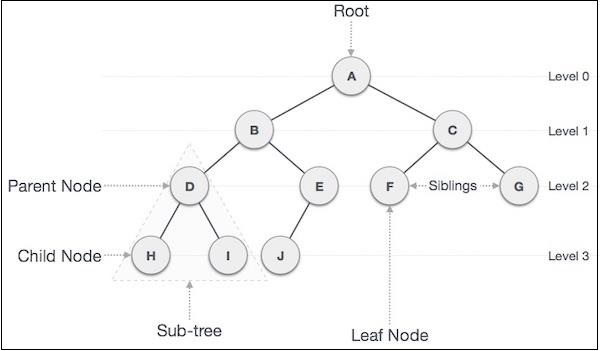# Binary Tree in Javascript

Binary Tree is a special data structure used for data storage purposes. A binary tree has a special condition that each node can have a maximum of two children. A binary tree has the benefits of both an ordered array and a linked list as search is as quick as in a sorted array and insertion or deletion operation are as fast as in the linked list.

Here is an illustration of a binary tree with some terms that we've discussed below −## Important Terms

Following are the important terms with respect to the tree.

• Path − Path refers to the sequence of nodes along the edges of a tree.

• Root − The node at the top of the tree is called root. There is only one root per tree and one path from the root node to any node.

• Parent − Any node except the root node has one edge upward to a node called the parent.

• Child − The node below a given node connected by its edge downward is called its child node.

• Leaf − The node which does not have any child node is called the leaf node.

• Subtree − Subtree represents the descendants of a node.

• Visiting − Visiting refers to checking the value of a node when control is on the node.

• Traversing − Traversing means passing through nodes in a specific order.

• Levels − Level of a node represents the generation of a node. If the root node is at level 0, then its next child node is at level 1, its grandchild is at level 2, and so on.

• Keys − Key represents a value of a node based on which a search operation is to be carried out for a node.